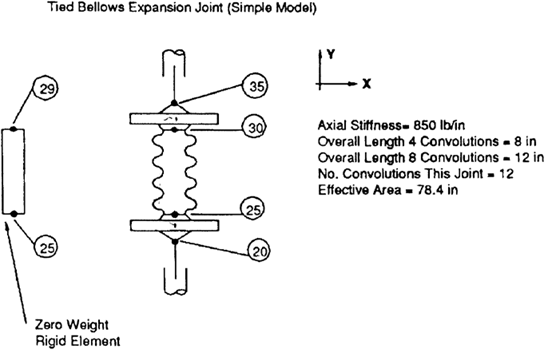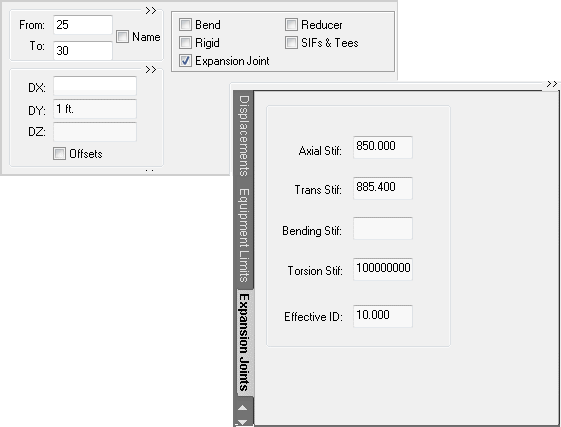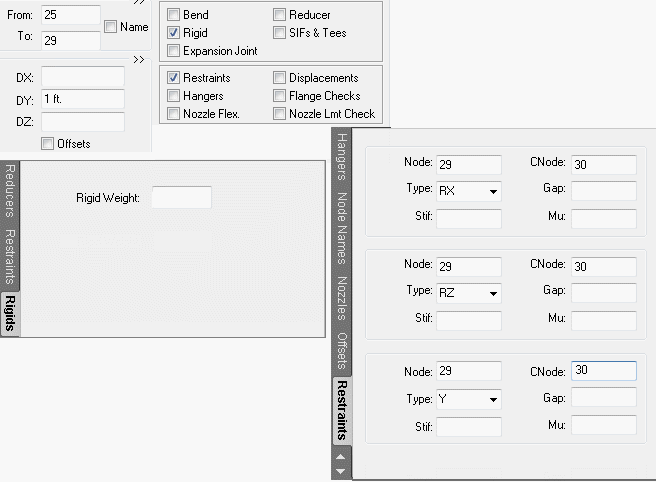# Tied Bellows Expansion Joint - Simple Model - CAESAR II - Reference Data

## CAESAR II Applications Guide (2019 Service Pack 1)

PPMProduct
CAESAR II
PPMCategory_custom
Reference Data
Version_CAESAR
11.0 (2019)

Calculate the lateral stiffness for the bellows. The flexible length, or convolution length, of the bellows is not listed in most expansion joint catalogs. The listed lengths include the rigid end pieces, such as flanges or pipe ends. Because the transverse stiffness is based on the flexible length, the flexible length must be known. A very simple way of pulling this value from the catalog is to examine the incremental increase in overall length of the joint as additional convolutions are added. With all convolutions the same length, this incremental length can be used to calculate the total flexible length.

In the following example, the total length of a four-convolution joint is 8-inches, and the total length of an 8-convolution joint is 12-inches. This means that the extra four convolutions add 4-inches, making the length of all twelve convolutions 12-inches. This also indicates that the rigid end pieces on this joint of four, eight, or twelve convolutions are 4-inches.

Deff = (4Aeff/p)1/2 = 10.0 in.

KTR = (3/2) (KAX) (Deff/L)2

L = Flexible Convolution Length = 12 in.

KTR = (3/2) (850) (10.0/12.0)2

= 885.4 lb/in.

Zero-weight rigid element (tie rod):Build the CAESAR II model of the flexible portion of the expansion joint. The rotational restraints between nodes 29 and 30 keep the two flanges parallel, which assumes three or more tie rods. In the field, the tie bars at four points around the expansion joint keep the flanges parallel.

The flanges and the tie bars form a parallelogram upon lateral deflection.